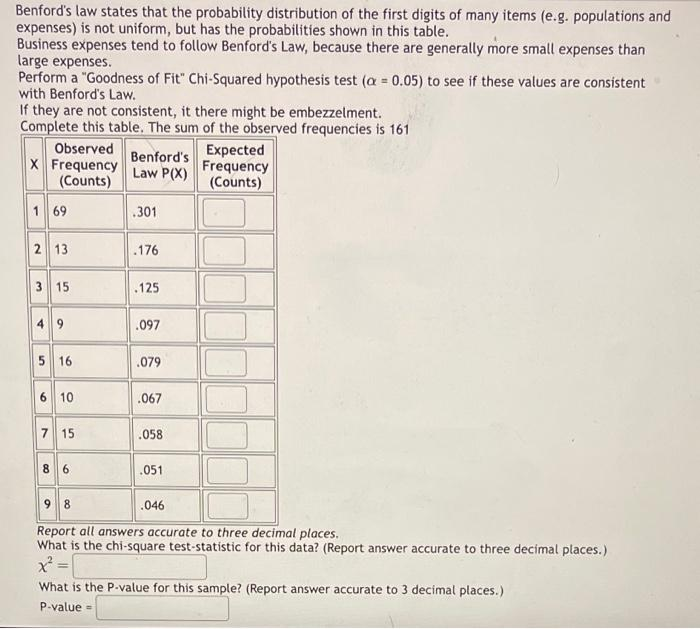Home / Expert Answers / Statistics and Probability / benford-39-s-law-states-that-the-probability-distribution-of-the-first-digits-of-many-items-e-g-popu-pa774

# (Solved): Benford's law states that the probability distribution of the first digits of many items (e.g. popu ...Benford's law states that the probability distribution of the first digits of many items (e.g. populations and expenses) is not uniform, but has the probabilities shown in this table. Business expenses tend to follow Benford's Law, because there are generally more small expenses than large expenses. Perform a "Goodness of Fit" Chi-Squared hypothesis test to see if these values are consistent with Benford's Law. If they are not consistent, it there might be embezzelment. Comolete this table. The sum of the nhserved frequencies is 161 Report all answers accurate to three decimal places. What is the chi-square test-statistic for this data? (Report answer accurate to three decimal places.) What is the P-value for this samole? (Report answer accurate to 3 decimal places.) -value

We have an Answer from Expert